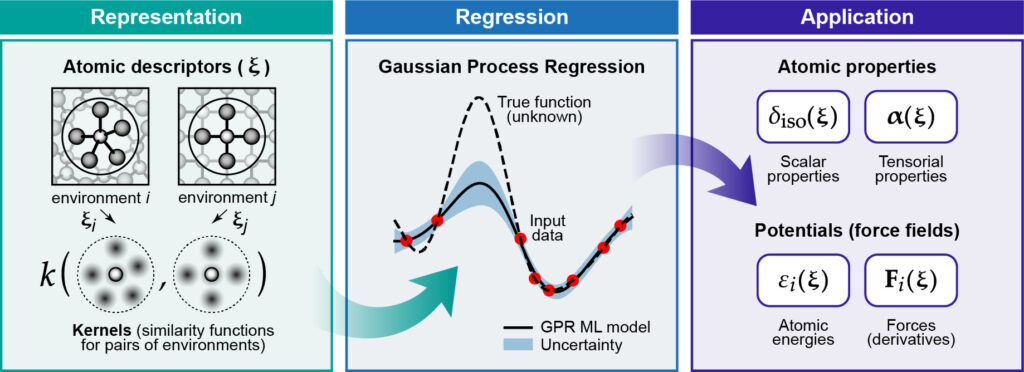# What’s GAP

The Gaussian approximation potential (GAP) is a theoretical/methodological framework for predicting the energy and forces in a system of interacting atoms. GAP learns the potential energy surface (PES) as a function of the atomic positions from precomputed reference (“training”) data, usually computed at the density functional theory (DFT) level of theory.The three basic steps in GAP modeling. Credit: Deringer et al., Chem. Rev. 121, 10073 (2021).

GAP performs a non-parametric kernel regression of the PES, estimating a local energy $\bar{\epsilon}_*$ (in its simplest implementation) as:

$\bar{\epsilon}_* = \delta^2 \sum\limits_{s = 1}^{N_s} \alpha_s k(*,s),$

where $*$ denotes the local atomic environment for which the prediction is being made, $\delta$ is a parameter defining the energy scale, $s$ runs over sparse set configurations (a subset of the training set), $\alpha_s$ are the fitting coefficients and $k(*,s)$ is a kernel – a measure of similarity between the local atomic environment $*$ and the atomic environment $s$ in the sparse set.

The usefulness of this approach is that we have rewritten the potential energy, a non-linear function of the atomic positions, $E = E(\{ \textbf{r}_i \})$, as a linear function in the kernels. This allows us to use linear algebra to obtain the fitting coefficients during the training stage.

Since GAP is not constrained to specific functional forms (like harmonic potentials) it can flexibly adapt to the training data, reproducing chemical phenomena like bond breaking and formation which are extremely challenging to capture with classical force fields.

The actual implementation of GAP includes many details beyond the simple expressions given above. These include the use of sophisticated atomic descriptors, sparsification strategies, database construction, adding locally parametrized force field, and software. To know more about GAP, we recommend the following literature:

• The original GAP paper: A.P. Bartók, M.C. Payne, R. Kondor, and G. Csányi. Gaussian approximation potentials: The accuracy of quantum mechanics, without the electrons. Phys. Rev. Lett. 104, 136403 (2010).
• Introduction of SOAP many-body descriptors: On representing chemical environments. A.P. Bartók, R. Kondor, and G. Csányi. Phys. Rev. B 87, 184115 (2013).
• An early review/tutorial paper expalining most of the currently employed GAP methodology: Gaussian approximation potentials: A brief tutorial introduction. A.P. Bartók and G. Csányi. Int. J. Quantum Chem. 115, 1051 (2015).
• A recent review paper: Gaussian Process Regression for Materials and Molecules. V.L. Deringer, A.P. Bartók, N. Bernstein, D.M. Wilkins, M. Ceriotti, and G. Csányi. Chem. Rev. 121, 10073 (2021).